# Chapter 1 Fitting Functions to Data

## 1.1  Exact fitting

### 1.1.1  Introduction

Suppose we have a set of real-number data pairs ${x}_{i},{y}_{i}, i=1,2,\dots N$. These can be considered to be a set of points in the $\mathit{xy}$-plane. They can also be thought of as a set of values $y$ of a function of $x$; see Fig. 1.1.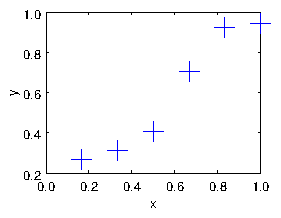Figure 1.1: Example of data to be fitted with a curve.
A frequent challenge is to find some kind of function that represents a "best fit" to the data in some sense. If the data were fitted perfectly, then clearly the function $f$ would have the property
 $f\left({x}_{i}\right)={y}_{i},\mathrm{ }\text{for all}i=1,\dots ,N.$ $\left(1.1\right)$
When the number of pairs is small and they are reasonably spaced out in $x$, then it may be reasonable to do an exact fit that satisfies this equation.

### 1.1.2  Representing an exact fitting function linearly

We have an infinite choice of possible fitting functions. Those functions must have a number of different adjustable parameters that are set so as to adjust the function to fit the data. One example is a polynomial.
 $f\left(x\right)={c}_{1}+{c}_{2}x+{c}_{3}{x}^{2}+...+{c}_{N}{x}^{N-1}$ $\left(1.2\right)$
Here the ${c}_{i}$ are the coefficients that must be adjusted to make the function fit the data. A polynomial whose coefficients are the adjustable parameters has a very useful property that it is linearly dependent upon the coefficients.
In order to fit eqs. (1.1) with the form of eq. (1.2) requires that $N$ simultaneous equations be satisfied. Those equations can be written as an $N×N$ matrix equation as follows:

 $\left(\begin{array}{ccccc}\hfill 1\hfill & \hfill {x}_{1}\hfill & \hfill {x}_{1}^{2}\hfill & \hfill ...\hfill & \hfill {x}_{1}^{N-1}\hfill \\ \hfill 1\hfill & \hfill {x}_{2}\hfill & \hfill {x}_{2}^{2}\hfill & \hfill ...\hfill & \hfill {x}_{2}^{N-1}\hfill \\ \hfill ...\hfill \\ \hfill 1\hfill & \hfill {x}_{N}\hfill & \hfill {x}_{N}^{2}\hfill & \hfill ...\hfill & \hfill {x}_{N}^{N-1}\hfill \end{array}\right)\left(\begin{array}{c}\hfill {c}_{1}\hfill \\ \hfill {c}_{2}\hfill \\ \hfill ...\hfill \\ \hfill {c}_{N}\hfill \end{array}\right)=\left(\begin{array}{c}\hfill {y}_{1}\hfill \\ \hfill {y}_{2}\hfill \\ \hfill ...\hfill \\ \hfill {y}_{N}\hfill \end{array}\right)$ $\left(1.3\right)$
Here we notice that in order for this to be a square matrix system we need the number of coefficients to be equal to the number of data pairs, $N$.
We also see that we could have used any set of $N$ functions ${f}_{i}$ as fitting functions, and written the representation:
 $f\left(x\right)={c}_{1}{f}_{1}\left(x\right)+{c}_{2}{f}_{2}\left(x\right)+{c}_{3}{f}_{3}\left(x\right)+...+{c}_{N}{f}_{N}\left(x\right)$ $\left(1.4\right)$
and then we would have obtained the matrix equation
 $\left(\begin{array}{ccccc}\hfill {f}_{1}\left({x}_{1}\right)\hfill & \hfill {f}_{2}\left({x}_{1}\right)\hfill & \hfill {f}_{3}\left({x}_{1}\right)\hfill & \hfill ...\hfill & \hfill {f}_{N}\left({x}_{1}\right)\hfill \\ \hfill {f}_{1}\left({x}_{2}\right)\hfill & \hfill {f}_{2}\left({x}_{2}\right)\hfill & \hfill {f}_{3}\left({x}_{2}\right)\hfill & \hfill ...\hfill & \hfill {f}_{N}\left({x}_{2}\right)\hfill \\ \hfill ...\hfill \\ \hfill {f}_{1}\left({x}_{N}\right)\hfill & \hfill {f}_{2}\left({x}_{N}\right)\hfill & \hfill {f}_{3}\left({x}_{N}\right)\hfill & \hfill ...\hfill & \hfill {f}_{N}\left({x}_{N}\right)\hfill \end{array}\right)\left(\begin{array}{c}\hfill {c}_{1}\hfill \\ \hfill {c}_{2}\hfill \\ \hfill ...\hfill \\ \hfill {c}_{N}\hfill \end{array}\right)=\left(\begin{array}{c}\hfill {y}_{1}\hfill \\ \hfill {y}_{2}\hfill \\ \hfill ...\hfill \\ \hfill {y}_{N}\hfill \end{array}\right)$ $\left(1.5\right)$
This is the most general form of representation of a fitting function that varies linearly with the unknown coefficients. The matrix${}^{1}$ we will call $\mathbf{S}$. It has elements ${S}_{\mathit{ij}}={f}_{j}\left({x}_{i}\right)$

### 1.1.3  Solving for the coefficients

When we have a matrix equation of the form $\mathbf{Sc}\mathbf{=}\mathbf{y}$, where $\mathbf{S}$ is a square matrix, then provided that the matrix is non-singular, that is, provided its determinant is non-zero, $|\mathbf{S}|\ne 0$, it possesses an inverse ${\mathbf{S}}^{-1}$. Multiplying on the left by this inverse we get:
 ${\mathbf{S}}^{-1}\mathbf{S}\mathbf{c}=\mathbf{c}={\mathbf{S}}^{-1}\mathbf{y}.$ $\left(1.6\right)$
In other words, we can solve for $\mathbf{c}$, the unknown coefficients, by inverting the function matrix, and multiplying the values to be fitted, $\mathbf{y}$ by that inverse.
Once we have the values of $\mathbf{c}$ we can evaluate the function $f\left(x\right)$ (eq. 1.2) at any $x$-value we like. Fig. 1.2 shows the result of fitting a 5th order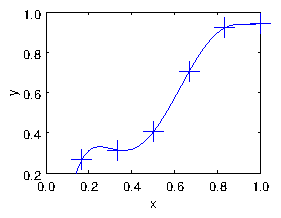Figure 1.2: Result of the polynomial fit.
polynomial (with 6 terms including the 1) to the six points of our data. The line goes exactly through every point. But there's a significant problem that the line is unconvincingly curvy near its ends${}^{2}$. It's not a terribly good fit.

## 1.2  Approximate Fitting

If we have lots of data which has scatter in it, arising from uncertainties or noise, then we almost certainly do not want to fit a curve so that it goes exactly through every point. For example see Fig. 1.3.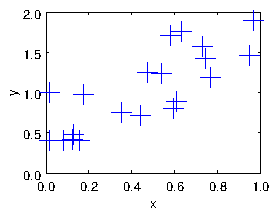Figure 1.3: A cloud of points with uncertainties and noise, to be fitted with a function.
What do we do then? Well, it turns out that we can use almost exactly the same approach, except with different number of points ($N$) and terms ($M$) in our linear fit. In other words we use a representation
 $f\left(x\right)={c}_{1}{f}_{1}\left(x\right)+{c}_{2}{f}_{2}\left(x\right)+{c}_{3}{f}_{3}\left(x\right)+...+{c}_{M}{f}_{M}\left(x\right)$ $\left(1.7\right)$
in which usually $M. We know now that we can't fit the data exactly. The set of equations we would have to satisfy to do so would be
 $\left(\begin{array}{ccccc}\hfill {f}_{1}\left({x}_{1}\right)\hfill & \hfill {f}_{2}\left({x}_{1}\right)\hfill & \hfill {f}_{3}\left({x}_{1}\right)\hfill & \hfill ...\hfill & \hfill {f}_{M}\left({x}_{1}\right)\hfill \\ \hfill {f}_{1}\left({x}_{2}\right)\hfill & \hfill {f}_{2}\left({x}_{2}\right)\hfill & \hfill {f}_{3}\left({x}_{2}\right)\hfill & \hfill ...\hfill & \hfill {f}_{M}\left({x}_{2}\right)\hfill \\ \hfill ...\hfill \\ \hfill {f}_{1}\left({x}_{N}\right)\hfill & \hfill {f}_{2}\left({x}_{N}\right)\hfill & \hfill {f}_{3}\left({x}_{N}\right)\hfill & \hfill ...\hfill & \hfill {f}_{M}\left({x}_{N}\right)\hfill \end{array}\right)\left(\begin{array}{c}\hfill {c}_{1}\hfill \\ \hfill {c}_{2}\hfill \\ \hfill ...\hfill \\ \hfill {c}_{M}\hfill \end{array}\right)=\left(\begin{array}{c}\hfill {y}_{1}\hfill \\ \hfill {y}_{2}\hfill \\ \hfill ...\hfill \\ \hfill {y}_{N}\hfill \end{array}\right)$ $\left(1.8\right)$
in which the function matrix $\mathbf{S}$ is now not square but has dimensions $N×M$. There are not enough coefficients ${c}_{j}$ to be able to satisfy these equations exactly. They are over-specified. Moreover, a non-square matrix doesn't have an inverse.
But we are not interested in fitting this data exactly. We want to fit some sort of line through the points that best-fits them.

### 1.2.1  Linear Least Squares

What do we mean by "best fit"? Especially when fitting a function of the linear form eq. (1.7), we usually mean that we want to minimize the vertical distance between the points and the line. If we had a fitted function $f\left(x\right)$, then for each data pair $\left({x}_{i},{y}_{i}\right)$, the square of the vertical distance between the line and the point is $\left({y}_{i}-f\left({x}_{i}{\right)\right)}^{2}$. So the sum, over all the points, of the square distance from the line is
 ${\mathit{\chi }}^{2}=\sum _{i=1,N}\left({y}_{i}-f\left({x}_{i}{\right)\right)}^{2}.$ $\left(1.9\right)$
We use the square of the distances in part because they are always positive. We don't want to add positive and negative distances, because a negative distance is just as bad as a positive one and we don't want them to cancel out. We generally call ${\mathit{\chi }}^{2}$ the "residual", or more simply the "chi-squared". It is an inverse measure of goodness of fit. The smaller it is the better. A linear least squares problem is: find the coefficients of our function $f$ that minimize the residual ${\mathit{\chi }}^{2}$.

### 1.2.2  SVD and the Moore-Penrose Pseudo-inverse

We seem to have gone off in a different direction from our original way to solve for the fitting coefficients by inverting the square matrix $\mathbf{S}$. How is that related to the finding of the least-squares solution to the over-specified set of equations (1.8)?
The answer is a piece of matrix magic! It turns out that there is (contrary to what one is taught in an elementary matrix course) a way to define the inverse of a non-square matrix or of a singular square matrix. It is called the (Moore-Penrose) pseudo-inverse. And once found it can be used in essentially exactly the way we did for the non-singular square matrix in the earlier treatment. That is, we solve for the coefficients using $\mathbf{c}={\mathbf{S}}^{-1}\mathbf{y}$, except that ${\mathbf{S}}^{-1}$ is now the pseudo-inverse.
The pseudo-inverse is best understood from a consideration of what is called the Singular Value Decomposition (SVD) of a matrix. This is the embodiment of a theorem in matrix mathematics that states that any $N×M$ matrix can always be expressed as the product of three other matrices with very special properties. For our $N×M$ matrix $\mathbf{S}$ this expression is:
 $\mathbf{S}=\mathbf{U}\mathbf{D}{\mathbf{V}}^{T},$ $\left(1.10\right)$
where ${}^{T}$ denotes transpose, and
• $\mathbf{U}$ is an $N×N$ orthonormal matrix
• $\mathbf{V}$ is an $M×M$ orthonormal matrix
• $\mathbf{D}$ is an $N×M$ diagonal matrix
Orthonormal${}^{3}$ means that the dot product of any column (regarded as a vector) with any other column is zero, and the dot product of a column with itself is unity. The inverse of an orthonormal matrix is its transpose. So
 $\underset{N×N}{\underset{⏟}{{\mathbf{U}}^{T}}}\underset{N×N}{\underset{⏟}{\mathbf{U}}}=\underset{N×N}{\underset{⏟}{\mathbf{I}}}\mathrm{ }\text{and}\mathrm{ }\underset{M×M}{\underset{⏟}{{\mathbf{V}}^{T}}}\underset{M×M}{\underset{⏟}{\mathbf{V}}}=\underset{M×M}{\underset{⏟}{\mathbf{I}}}$ $\left(1.11\right)$
A diagonal matrix has non-zero elements only on the diagonal. But if it is non-square, as it is if $M, then it is padded with extra rows of zeros (or extra columns if $N).
 $\mathbf{D}=\left(\begin{array}{ccccc}\hfill {d}_{1}\hfill & \hfill 0\hfill & \hfill 0\hfill & \hfill \dots \hfill & \hfill 0\hfill \\ \hfill 0\hfill & \hfill {d}_{2}\hfill & \hfill 0\hfill & \hfill \hfill & \hfill \hfill \\ \hfill 0\hfill & \hfill \hfill & \hfill \ddots \hfill & \hfill \hfill & \hfill \hfill \\ \hfill :\hfill & \hfill \hfill & \hfill \hfill & \hfill \ddots \hfill & \hfill 0\hfill \\ \hfill \hfill & \hfill \hfill & \hfill 0\hfill & \hfill 0\hfill & \hfill {d}_{M}\hfill \\ \hfill 0\hfill & \hfill 0\hfill & \hfill 0\hfill & \hfill 0\hfill & \hfill 0\hfill \end{array}\right)$ $\left(1.12\right)$
A sense of what the SVD is can be gained from by thinking${}^{4}$ in terms of the eigenanalysis of the matrix ${\mathbf{S}}^{T}\mathbf{S}$. Its eigenvalues are ${d}_{i}^{2}$.
The pseudo-inverse can be considered to be
 ${\mathbf{S}}^{-1}=\mathbf{V}{\mathbf{D}}^{-1}{\mathbf{U}}^{T}.$ $\left(1.13\right)$
Here ${\mathbf{D}}^{-1}$ is a $M×N$ diagonal matrix whose entries are the inverse of those of $\mathbf{D}$, i.e. $1/{d}_{j}$:
 ${\mathbf{D}}^{-1}=\left(\begin{array}{cccccc}\hfill 1/{d}_{1}\hfill & \hfill 0\hfill & \hfill 0\hfill & \hfill \dots \hfill & \hfill 0\hfill & \hfill 0\hfill \\ \hfill 0\hfill & \hfill 1/{d}_{2}\hfill & \hfill 0\hfill & \hfill \hfill & \hfill \hfill & \hfill 0\hfill \\ \hfill 0\hfill & \hfill \hfill & \hfill \ddots \hfill & \hfill \hfill & \hfill \hfill & \hfill 0\hfill \\ \hfill :\hfill & \hfill \hfill & \hfill \hfill & \hfill \ddots \hfill & \hfill 0\hfill & \hfill 0\hfill \\ \hfill 0\hfill & \hfill \dots \hfill & \hfill 0\hfill & \hfill 0\hfill & \hfill 1/{d}_{M}\hfill & \hfill 0\hfill \end{array}\right).$ $\left(1.14\right)$
It's clear that eq. (1.13) is in some sense an inverse of $\mathbf{S}$, because formally
 ${\mathbf{S}}^{-1}\mathbf{S}=\left(\mathbf{V}{\mathbf{D}}^{-1}{\mathbf{U}}^{T}\right)\left(\mathbf{U}\mathbf{D}{\mathbf{V}}^{T}\right)=\mathbf{V}{\mathbf{D}}^{-1}\mathbf{D}{\mathbf{V}}^{T}=\mathbf{V}{\mathbf{V}}^{T}=\mathbf{I}.$ $\left(1.15\right)$
If $M\le N$ and none of the ${d}_{j}$ is zero, then all the operations in this matrix multiplication reduction are valid, because
 $\underset{M×N}{\underset{⏟}{{\mathbf{D}}^{-1}}}\underset{N×M}{\underset{⏟}{\mathbf{D}}}=\underset{M×M}{\underset{⏟}{\mathbf{I}}}.$ $\left(1.16\right)$
But see the enrichment section${}^{5}$ for detailed discussion of other cases.
The most important thing for our present purposes is that if $M\le N$ then we can find a solution of the over-specified (rectangular matrix) fitting problem $\mathbf{S}\mathbf{c}=\mathbf{y}$ as $\mathbf{c}={\mathbf{S}}^{-1}\mathbf{y}$, using the pseudo-inverse. The set of coefficients $\mathbf{c}$ we get corresponds to more than one possible set of ${y}_{i}$-values, but that does not matter.
Also, one can show${}^{6}$, that the specific solution that is obtained by this matrix product is in fact the least squares solution for $\mathbf{c}$; i.e. the solution that minimizes the residual ${\mathit{\chi }}^{2}$. And if there is any freedom in the choice of $\mathbf{c}$, such that the residual is at its minimum for a range of different $\mathbf{c}$, then the solution which minimizes $|\mathbf{c}{|}^{2}$ is the one found.
The beauty of this fact is that one can implement a simple code, which calls a function pinv to find the pseudo-inverse, and it will work just fine if the matrix $\mathbf{S}$ is singular or even rectangular.
As a matter of computational efficiency, one should note that in Octave the backslash operator, is equivalent to multiplying by the pseudo-inverse (i.e. pinv(S)*y = S\y), but calculated far more efficiently${}^{7}$. So backslash is preferable in computationally costly code, because it is roughly 5 times faster. You probably won't notice the difference for matrix dimensions smaller than a few hundred.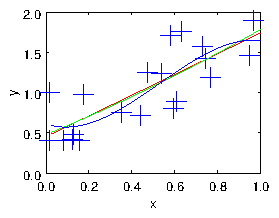Figure 1.4: The cloud of points fitted with linear, quadratic, and cubic polynomials.

### 1.2.3  Smoothing and Regularization

As we illustrate in Fig. 1.4, by choosing the number of degrees of freedom of the fitting function one can adjust the smoothness of the fit to the data. However, the choice of basis functions then constrains one in a way that has been pre-specified. It might not in fact be the best way to smooth the data to fit it by (say) a straight line or a parabola.
A better way to smooth is by "regularization" in which we add some measure of roughness to the residual we are seeking to minimize. The roughness (which is the inverse of the smoothness) is a measure of how wiggly the fit line is. It can in principle be pretty much anything that can be written in the form of a matrix times the fit coefficients. I'll give an example in a moment. Let's assume the roughness measure is homogeneous, in the sense that we are trying to make it as near zero as possible. Such a target would be $\mathbf{R}\mathbf{c}=0$, where $\mathbf{R}$ is a matrix of dimension ${N}_{R}×M$, where ${N}_{R}$ is the number of distinct roughness constraints. Presumably we can't satisfy this equation perfectly because a fully smooth function would have no variation, and be unable to fit the data. But we want to minimize the square of the roughness $\left(\mathbf{R}\mathbf{c}{\right)}^{T}\mathbf{R}\mathbf{c}$. We can try to fulfil the requirement to fit the data, and to minimize the roughness, in a least-squares sense by constructing an expanded compound matrix system combining the original equations and the regularization, thus${}^{8}$
 $\left(\begin{array}{c}\hfill \mathbf{S}\hfill \\ \hfill \mathit{\lambda }\mathbf{R}\hfill \end{array}\right)\mathbf{c}=\left(\begin{array}{c}\hfill \mathbf{y}\hfill \\ \hfill 0\hfill \end{array}\right).$ $\left(1.17\right)$
If we solve this system in a least-squares sense by using the pseudo inverse of the compound matrix $\left(\genfrac{}{}{0}{}{\mathbf{S}}{\mathit{\lambda }\mathbf{R}}\right)$, then we will have found the coefficients that "best" make the roughness zero as well as fitting the data: in the sense that the total residual
 ${\mathit{\chi }}^{2}=\sum _{i=1,N}\left({y}_{i}-f\left({x}_{i}{\right)\right)}^{2}+{\mathit{\lambda }}^{2}\sum _{k=1,{N}_{R}}\left(\sum _{j}{R}_{\mathit{kj}}{c}_{j}{\right)}^{2}$ $\left(1.18\right)$
is minimized. The value of $\mathit{\lambda }$ controls the weight of the smoothing. If it is large, then we prefer smoother solutions. If it is small or zero we do negligible smoothing.
As a specific one-dimensional example, we might decide that the roughness we want to minimize is represented by the second derivative of the function: ${d}^{2}f/{\mathit{dx}}^{2}$. Making this quantity on average small has the effect of minimizing the wiggles in the function, so it is an appropriate roughness measure. We could therefore choose $\mathbf{R}$ such that it represented that derivative at a set of chosen points ${x}_{k}, k=1,{N}_{R}$ (not the same as the data points ${x}_{i}$) in which case:
 ${R}_{\mathit{kj}}={\frac{{d}^{2}{f}_{j}}{{\mathit{dx}}^{2}}|}_{{x}_{k}}.$ $\left(1.19\right)$
The ${x}_{k}$ might, for example, be equally spaced over the $x$-interval of interest, in which case${}^{9}$ the squared roughness measure could be considered a discrete approximation to the integral, over the interval, of the quantity $\left({d}^{2}f/{\mathit{dx}}^{2}{\right)}^{2}$.

## 1.3  Tomographic Image Reconstruction

Consider the problem of x-ray tomography. We make many measurements of the integrated density of matter along chords in a plane section through some object whose interior we wish to reconstruct. These are generally done by measuring the attenuation of x-rays along each chord, but the mathematical technique is independent of the physics. We seek a representation of the density of the object in the form
 $\mathit{\rho }\left(x,y\right)=\sum _{j=1,M}{c}_{j}{\mathit{\rho }}_{j}\left(x,y\right),$ $\left(1.20\right)$
where ${\mathit{\rho }}_{j}\left(x,y\right)$ are basis functions over the plane. They might actually be as simple as pixels over mesh ${x}_{k}$ and ${y}_{l}$, such that ${\mathit{\rho }}_{j}\left(x,y\right)\to {\mathit{\rho }}_{\mathit{kl}}\left(x,y\right)=1$ when ${x}_{k} and ${y}_{l}, and zero otherwise. However, the form of basis function that won Alan Cormack the Nobel prize for medicine in his implementation of "computerized tomography" (the CT scan) was much more cleverly chosen to build the smoothing into the basis functions. Be careful thinking about multidimensional fitting. For constructing fitting matrices, the list of basis functions should be considered to be logically arranged from 1 to $M$ in a single index $j$ so that the coefficients are a single column vector. But the physical arrangement of the basis functions might more naturally be expressed using two indices $k,l$ referring to the different spatial dimensions. If so then they must be mapped in some consistent manner to the vector column.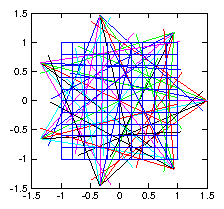Figure 1.5: Illustrative layout of tomographic reconstruction of density in a plane using multiple fans of chordal observations.
Each chord along which measurements are made, passes through the basis functions (e.g. the pixels), and for a particular set of coefficients ${c}_{j}$ we therefore get a chordal measurement value
 ${v}_{i}={\int }_{{l}_{i}}\mathit{\rho }d\mathit{\ell }={\int }_{{l}_{i}}\sum _{j=1,M}{c}_{j}{\mathit{\rho }}_{j}\left(x,y\right)d\mathit{\ell }=\sum _{j=1,M}{\int }_{{l}_{i}}{\mathit{\rho }}_{j}\left(x,y\right)d\mathit{\ell } {c}_{j}=\mathbf{S}\mathbf{c},$ $\left(1.21\right)$
where the $N×M$ matrix $\mathbf{S}$ is formed from the integrals along each of the $N$ lines of sight ${l}_{i}$, so that ${S}_{\mathit{ij}}={\int }_{{l}_{i}}{\mathit{\rho }}_{j}\left(x,y\right)d\mathit{\ell }$. It represents the contribution of basis function $j$ to measurement $i$. Our fitting problem is thus rendered into the standard form:
 $\mathbf{S}\mathbf{c}=\mathbf{v},$ $\left(1.22\right)$
in which a rather large number $M$ of basis functions might be involved. We can solve this by pseudo-inverse: $\mathbf{c}={\mathbf{S}}^{-1}\mathbf{v}$, and if the system is overdetermined, such that the effective number of different chords is larger than the number of basis functions, it will probably work.
The problem is, however, usually under-determined, in the sense that we don't really have enough independent chordal measurements to determine the density in each pixel (for example). This is true even if we apparently have more measurements than pixels, because generally there is a finite noise or uncertainty level in the chordal measurements that becomes amplified by the inversion process. This is illustrated by a simple test as shown in Fig. 1.6.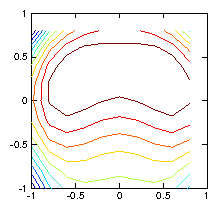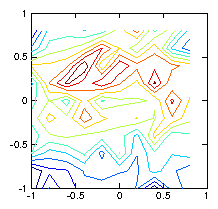Figure 1.6: Contour plots of the initial test $\mathit{\rho }$-function (left) used to calculate the chordal integrals, and its reconstruction based upon inversion of the chordal data (right). The number of pixels (100) exceeds the number of views (49), and the number of singular values used in the pseudo inverse is restricted to 30. Still they do not agree well, because various artifacts appear. Reducing the number of singular values does not help.
We then almost certainly want to smooth the representation otherwise all sorts of meaningless artifacts will appear in our reconstruction that have no physical existence. If we try to do this by forming a pseudo-inverse in which a smaller number of singular values are retained, and the others put to zero, there is no guarantee that this will get rid of the roughness. Fig. 1.6 gives an example.
If we instead smooth the reconstruction by regularization, using as our measure of roughness the discrete (2-D) Laplacian (${\nabla }^{2}\mathit{\rho }$) evaluated at each pixel. We get a far better result, as shown in Fig. 1.7. It turns out that this good result is rather insensitive to the value of ${\mathit{\lambda }}^{2}$ over two or three orders of magnitude.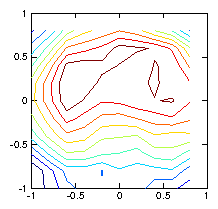Figure 1.7: Reconstruction using a regularization smoothing based upon ${\nabla }^{2}\mathit{\rho }$. The contours are much nearer to reality.

## 1.4  Efficiency and Nonlinearity

Using the inverse or pseudo-inverse to solve for the coefficients of a fitting function is intuitive and straight-forward. However, in many cases it is not the most computationally efficient approach. For moderate size problems, modern computers have more than enough power to overcome the inefficiencies, but in a situation with multiple dimensions, such as tomography, it is easy for the matrix that needs to be inverted to become enormous, because that matrix's side length is the total number of pixels or elements in the fit, which may be, for example, the product of the side lengths nx$×$ny. The giant matrix that has to be inverted, may be very "sparse", meaning that all but a very few of its elements are zero. It can then become overwhelming in terms of storage and cpu to use the direct inversion methods we have discussed here. We'll see other approaches later.
Some fitting problems are nonlinear. For example, suppose one had a photon spectrum of a particular spectral line to which one wished to fit a Gaussian function of particular center, width, and height. That's a problem that cannot be expressed as a linear sum of functions. In that case fitting becomes more elaborate${}^{10}$, and less reliable. There are some potted fitting programs out there, but it's usually better if you can avoid them.

## Worked Example: Fitting sinusoidal functions

Suppose we wish to fit a set of data ${x}_{i},{y}_{i}$ spread over the range of independent variable $a\le x\le b$. And suppose we know the function is zero at the boundaries of the range, at $x=a$ and $x=b$. It makes sense to incorporate our knowledge of the boundary values into the choice of functions to fit, and choose those functions ${f}_{n}$ to be zero at $x=a$ and $x=b$. There are numerous well known sets of functions that have the property of being zero at two separated points. The points where standard functions are zero are of course not some arbitrary $a$ and $b$. But we can scale the independent variable $x$ so that $a$ and $b$ are mapped to the appropriate points for any choice of function set.
Suppose the functions that we decide to use for fitting are sinusoids${}^{11}$: ${f}_{n}=\mathrm{sin}\left(n\mathit{\theta }\right)$ all of which are zero at $\mathit{\theta }=0$ and $\mathit{\theta }=\mathit{\pi }$. We can make this set fit our $x$ range by using the scaling
 $\mathit{\theta }=\mathit{\pi }\left(x-a\right)/\left(b-a\right),$ $\left(1.23\right)$
so that $\mathit{\theta }$ ranges from 0 to $\mathit{\pi }$ as $x$ ranges from $a$ to $b$. Now we want to find the best fit to our data in the form
 $f\left(x\right)={c}_{1}\mathrm{sin}\left(\mathit{\theta }\right)+{c}_{2}\mathrm{sin}\left(2\mathit{\theta }\right)+{c}_{3}\mathrm{sin}\left(3\mathit{\theta }\right)+\dots +{c}_{M}\mathrm{sin}\left(M\mathit{\theta }\right).$ $\left(1.24\right)$
We therefore want the least-squares solution for the ${c}_{i}$ of
 $\mathbf{S}\mathbf{c}=\left(\begin{array}{cccc}\hfill \mathrm{sin}\left(1{\mathit{\theta }}_{1}\right)\hfill & \hfill \mathrm{sin}\left(2{\mathit{\theta }}_{1}\right)\hfill & \hfill ...\hfill & \hfill \mathrm{sin}\left(M{\mathit{\theta }}_{1}\right)\hfill \\ \hfill \mathrm{sin}\left(1{\mathit{\theta }}_{2}\right)\hfill & \hfill \mathrm{sin}\left(2{\mathit{\theta }}_{2}\right)\hfill & \hfill ...\hfill & \hfill \mathrm{sin}\left(M{\mathit{\theta }}_{2}\right)\hfill \\ \hfill ...\hfill \\ \hfill \mathrm{sin}\left(1{\mathit{\theta }}_{N}\right)\hfill & \hfill \mathrm{sin}\left(2{\mathit{\theta }}_{N}\right)\hfill & \hfill ...\hfill & \hfill \mathrm{sin}\left(M{\mathit{\theta }}_{N}\right)\hfill \end{array}\right)\left(\begin{array}{c}\hfill {c}_{1}\hfill \\ \hfill {c}_{2}\hfill \\ \hfill ...\hfill \\ \hfill {c}_{M}\hfill \end{array}\right)=\left(\begin{array}{c}\hfill {y}_{1}\hfill \\ \hfill {y}_{2}\hfill \\ \hfill ...\hfill \\ \hfill {y}_{N}\hfill \end{array}\right)=\mathbf{y}$ $\left(1.25\right)$
We find this solution by the following procedure.
1. If necessary, construct column vectors $\mathbf{x}$ and $\mathbf{y}$ from the data.
2. Calculate the scaled vector $\mathbit{\theta }$ from $\mathbf{x}$.
3. Construct the matrix $\mathbf{S}$ whose $\mathit{ij}$th entry is $\mathrm{sin}\left(j{\mathit{\theta }}_{i}\right)$
4. Least-squares-solve $\mathbf{S}\mathbf{c}=\mathbf{y}$ (e.g. by pseudo-inverse) to find $\mathbf{c}$.
5. Evaluate the fit at any $x$ by substituting the expression for $\mathit{\theta }$, 1.23, into 1.24.
This process may be programmed in a mathematical system like Matlab or Octave, which has built-in matrix multiplication, very concisely${}^{12}$ as follows (entries following % are comments).
```  % Suppose x and y exist as column vectors of length N. (Nx1 matrices)
j=[1:M];               % Create a 1xM matrix containing numbers 1 to M.
theta=pi*(x-a)/(b-a);  % Scale x to obtain the column vector theta.
S=sin(theta*j);        % Construct the matrix S using an outer product.
Sinv=pinv(S);          % Pseudo invert it.
c=Sinv*y;              % Matrix multiply y to find the coefficients c.

```
The fit can then be evaluated for any $x$ value (or array) xfit, in the form effectively of a scalar product of $\mathrm{sin}\left(\mathit{\theta }\mathbf{j}\right)$ with $\mathbf{c}$. The code is likewise astonishingly brief, and will need careful thought (especially noting what the dimensions of the matrices are) to understand what is actually happening.
```yfit=sin(pi*(xfit-a)/(b-a)*j)*c; % Evaluate the yfit at any xfit

```
An example is shown in Fig. 1.8.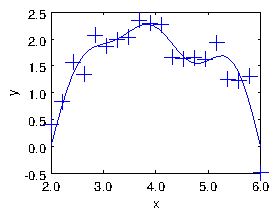Figure 1.8: The result of the fit of sinusoids up to $M=5$ to a noisy dataset of size $N=20$. The points are the input data. The curve is constructed by using the yfit expression on an xfit array of some convenient length spanning the $x$-range, and then simply plotting yfit versus xfit.

## Exercise 1. Data fitting

1. Given a set of $N$ values ${y}_{i}$ of a function $y\left(x\right)$ at the positions ${x}_{i}$, write a short code to fit a polynomial having order one less than $N$ (so there are $N$ coefficients of the polynomial) to the data.
Obtain a set of $\left(N=\right)$ 6 numbers from
http://silas.psfc.mit.edu/22.15/15numbers.html
(or if that is not accessible use ${y}_{i}=\left[0.892,1.44,1.31,1.66,1.10,1.19\right]$). Take the values ${y}_{i}$ to be at the positions ${x}_{i}=\left[0.0,0.2,0.4,0.6,0.8,1.0\right]$. Run your code on this data and find the coefficients ${c}_{j}$.
Plot together (on the same plot) the resulting fitted polynomial representing $y\left(x\right)$ (with sufficient resolution to give a smooth curve) and the original data points, over the domain $0\le x\le 1$.
Submit the following as your solution:
1. Your code in a computer format that is capable of being executed.
2. The numeric values of your coefficients ${c}_{j}, j=1,N$.
4. Brief commentary ($<300$ words) on what problems you faced and how you solved them.

2. Save your code from part 1. Make a copy of it with a new name and change the new code as needed to fit (in the linear least squares sense) a polynomial of order possibly lower than $N-1$ to a set of data ${x}_{i}$, ${y}_{i}$ (for which the points are in no particular order).
Obtain a pair of data sets of length $\left(N=\right)$ 20 numbers ${x}_{i}$, ${y}_{i}$ from the same URL by changing the entry in the "Number of Numbers" box. (Or if that is inaccessible, generate your own data set from random numbers added to a line.) Run your code on that data to produce the fitting coefficients ${c}_{j}$ when the number of coefficients of the polynomial is ($M=$) (a) 1, (b) 2, (c) 3. That is: constant, linear, quadratic.
Plot the fitted curves and the original data points on the same plot(s) for all three cases.
Submit the following as your solution:
1. Your code in a computer format that is capable of being executed.
2. Your coefficients ${c}_{j}, j=1,M$, for three cases (a), (b), (c).